# Ratio Test Example with an Exponent

Previous: A Simple Ratio Test Example

Next: Videos on The Ratio Test

## Question

Using only the ratio test, determine whether or not the series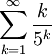converges, diverges, or yields no conclusion.

## Complete Solution

Applying the ratio test yieldsSince the limit equals, the ratio test tells us that the series converges.

## Explanation of Each Step

### Step (1)

To apply the ratio test, we must evaluate the limit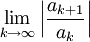In our problem, we have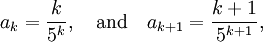and we substitute them into our limit.

### Step (2)

In Step (2), we use a little algebraic manipulation to make things easier to look at### Step (3)

Step (3) uses a property of absolute values. Recall that for real numbersand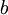,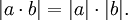### Step (4)

Step (4) uses a property of limits values. Recall that for functions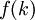and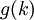,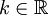that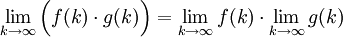### Step (5)

Here we evaluate a limit: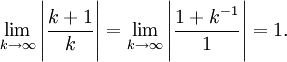### Step (6)

Some algebraic manipulation helps us see how we can simplify our problem. Recall that, using laws of exponentials, that for real numbers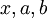, that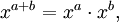so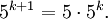Previous: A Simple Ratio Test Example

Next: Videos on The Ratio Test